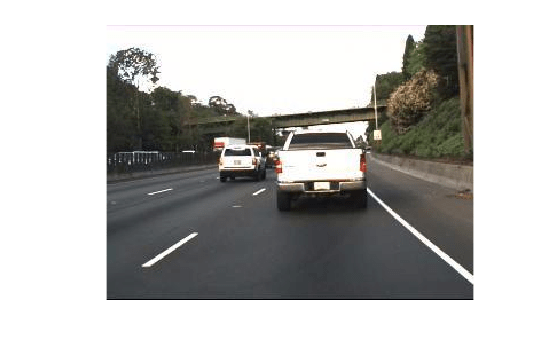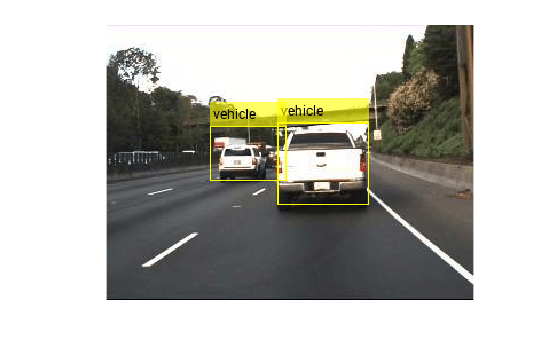# detect

Detect objects using Fast R-CNN object detector

## Syntax

``bboxes = detect(detector,I)``
``````[bboxes,scores] = detect(detector,I)``````
``[___,labels] = detect(detector,I)``
``[___] = detect(___,roi)``
``detectionResults = detect(detector,ds)``
``[___] = detect(___,Name,Value)``

## Description

````bboxes = detect(detector,I)` detects objects within a single image or an array of images, `I`, using a Fast R-CNN (regions with convolutional neural networks) object detector. The locations of objects detected are returned as a set of bounding boxes.When using this function, use of a CUDA® enabled NVIDIA® GPU is highly recommended. The GPU reduces computation time significantly. Usage of the GPU requires Parallel Computing Toolbox™. For information about the supported compute capabilities, see GPU Support by Release (Parallel Computing Toolbox).```
``````[bboxes,scores] = detect(detector,I)``` also returns the detection scores for each bounding box.```

example

````[___,labels] = detect(detector,I)` also returns a categorical array of labels assigned to the bounding boxes, using either of the preceding syntaxes. The labels used for object classes are defined during training using the `trainFastRCNNObjectDetector` function.```
````[___] = detect(___,roi)` detects objects within the rectangular search region specified by `roi`.```
````detectionResults = detect(detector,ds)` detects objects within the series of images returned by the `read` function of the input datastore.```
````[___] = detect(___,Name,Value)` specifies options using one or more `Name,Value` pair arguments. For example, `detect(detector,I,'NumStongestRegions',1000)` limits the number of strongest region proposals to 1000.```

## Examples

collapse all

Detect vehicles within an image by using a Faster R-CNN object detector.

Load a Faster R-CNN object detector pretrained to detect vehicles.

```data = load('fasterRCNNVehicleTrainingData.mat', 'detector'); detector = data.detector;```

```I = imread('highway.png'); imshow(I)```Run the detector on the image and inspect the results. The labels come from the `ClassNames` property of the detector.

`[bboxes,scores,labels] = detect(detector,I)`
```bboxes = 2×4 150 86 80 72 91 89 67 48 ```
```scores = 2x1 single column vector 1.0000 0.9001 ```
```labels = 2x1 categorical vehicle vehicle ```

The detector has high confidence in the detections. Annotate the image with the bounding boxes for the detections and the corresponding detection scores.

``` detectedI = insertObjectAnnotation(I,'Rectangle',bboxes,cellstr(labels)); figure imshow(detectedI)```## Input Arguments

collapse all

Fast R-CNN object detector, specified as a `fastRCNNObjectDetector` object. To create this object, call the `trainFastRCNNObjectDetector` function with training data as input.

Input image, specified as an H-by-W-by-C-by-B numeric array of images Images must be real, nonsparse, grayscale or RGB image.

• H: Height

• W: Width

• C: The channel size in each image must be equal to the network's input channel size. For example, for grayscale images, C must be equal to `1`. For RGB color images, it must be equal to `3`.

• B: The number of images in the array.

The detector is sensitive to the range of the input image. Therefore, ensure that the input image range is similar to the range of the images used to train the detector. For example, if the detector was trained on `uint8` images, rescale this input image to the range [0, 255] by using the `im2uint8` or `rescale` function. The size of this input image should be comparable to the sizes of the images used in training. If these sizes are very different, the detector has difficulty detecting objects because the scale of the objects in the input image differs from the scale of the objects the detector was trained to identify. Consider whether you used the `SmallestImageDimension` property during training to modify the size of training images.

Data Types: `uint8` | `uint16` | `int16` | `double` | `single` | `logical`

Datastore, specified as a datastore object containing a collection of images. Each image must be a grayscale, RGB, or multichannel image. The function processes only the first column of the datastore, which must contain images and must be cell arrays or tables with multiple columns.

Search region of interest, specified as an [x y width height] vector. The vector specifies the upper left corner and size of a region in pixels.

### Name-Value Arguments

Specify optional pairs of arguments as `Name1=Value1,...,NameN=ValueN`, where `Name` is the argument name and `Value` is the corresponding value. Name-value arguments must appear after other arguments, but the order of the pairs does not matter.

Before R2021a, use commas to separate each name and value, and enclose `Name` in quotes.

Example: `'NumStrongestRegions',1000`

Detection threshold, specified as a scalar in the range [0, 1]. Detections that have scores less than this threshold value are removed. To reduce false positives, increase this value.

Maximum number of strongest region proposals, specified as the comma-separated pair consisting of `'NumStrongestRegions'` and a positive integer. Reduce this value to speed up processing time at the cost of detection accuracy. To use all region proposals, specify this value as `Inf`.

Select the strongest bounding box for each detected object, specified as the comma-separated pair consisting of `'SelectStrongest'` and either `true` or `false`.

• `true` — Return the strongest bounding box per object. To select these boxes, `detect` calls the `selectStrongestBboxMulticlass` function, which uses nonmaximal suppression to eliminate overlapping bounding boxes based on their confidence scores.

For example:

``` selectStrongestBboxMulticlass(bbox,scores, ... 'RatioType','Min', ... 'OverlapThreshold',0.5);```

• `false` — Return all detected bounding boxes. You can then create your own custom operation to eliminate overlapping bounding boxes.

Minimum region size that contains a detected object, specified as the comma-separated pair consisting of `'MinSize'` and a [height width] vector. Units are in pixels.

By default, `MinSize` is the smallest object that the trained `detector` can detect.

Maximum region size that contains a detected object, specified as the comma-separated pair consisting of `'MaxSize'` and a [height width] vector. Units are in pixels.

To reduce computation time, set this value to the known maximum region size for the objects being detected in the image. By default, `'MaxSize'` is set to the height and width of the input image, `I`.

Minimum batch size, specified as the comma-separated pair consisting of `'MiniBatchSize'` and a scalar value. Use the `MiniBatchSize` to process a large collection of images. Images are grouped into minibatches and processed as a batch to improve computation efficiency. Increase the minibatch size to decrease processing time. Decrease the size to use less memory.

Hardware resource on which to run the detector, specified as the comma-separated pair consisting of `'ExecutionEnvironment'` and `'auto'`, `'gpu'`, or `'cpu'`.

• `'auto'` — Use a GPU if it is available. Otherwise, use the CPU.

• `'gpu'` — Use the GPU. To use a GPU, you must have Parallel Computing Toolbox and a CUDA enabled NVIDIA GPU. If a suitable GPU is not available, the function returns an error. For information about the supported compute capabilities, see GPU Support by Release (Parallel Computing Toolbox).

• `'cpu'` — Use the CPU.

## Output Arguments

collapse all

Location of objects detected within the input image or images, returned as an M-by-4 matrix or a B-by-1 cell array. M is the number of bounding boxes in an image, and B is the number of M-by-4 matrices when the input contains an array of images.

Each row of `bboxes` contains a four-element vector of the form [x y width height]. This vector specifies the upper left corner and size of that corresponding bounding box in pixels.

Detection confidence scores, returned as an M-by-1 vector or a B-by-1 cell array. M is the number of bounding boxes in an image, and B is the number of M-by-1 vectors when the input contains an array of images. A higher score indicates higher confidence in the detection.

Labels for bounding boxes, returned as an M-by-1 categorical array or a B-by-1 cell array. M is the number of labels in an image, and B is the number of M-by-1 categorical arrays when the input contains an array of images. You define the class names used to label the objects when you train the input `detector`.

Detection results, returned as a 3-column table with variable names, Boxes, Scores, and Labels. The Boxes column contains M-by-4 matrices, of M bounding boxes for the objects found in the image. Each row contains a bounding box as a 4-element vector in the format [x,y,width,height]. The format specifies the upper-left corner location and size in pixels of the bounding box in the corresponding image.

## Version History

Introduced in R2017a